# DFA to Regular Expression | Examples

## DFA to Regular Expression-

The two popular methods for converting a DFA to its regular expression are-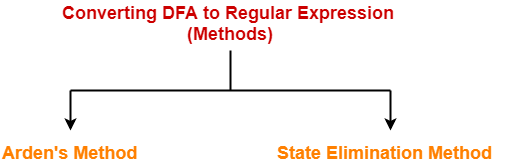1. Arden’s Method
2. State Elimination Method

## State Elimination Method-

This method involves the following steps in finding the regular expression for any given DFA-

### Thumb Rule

The initial state of the DFA must not have any incoming edge.

• If there exists any incoming edge to the initial state, then create a new initial state having no incoming edge to it.

### Example-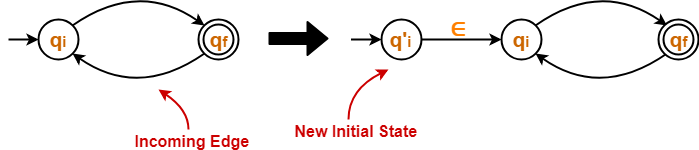### Thumb Rule

There must exist only one final state in the DFA.

• If there exists multiple final states in the DFA, then convert all the final states into non-final states and create a new single final state.

### Example-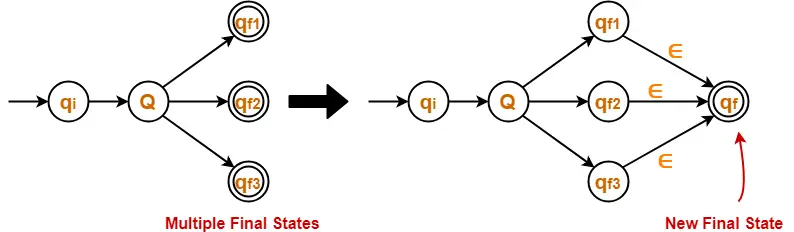### Thumb Rule

The final state of the DFA must not have any outgoing edge.

• If there exists any outgoing edge from the final state, then create a new final state having no outgoing edge from it.

### Example-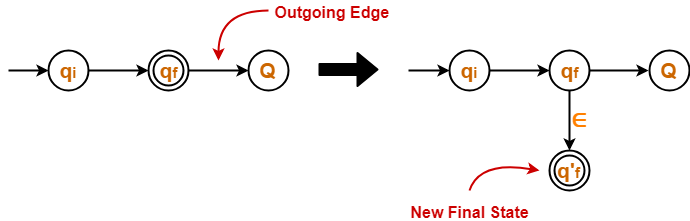### Step-04:

• Eliminate all the intermediate states one by one.
• These states may be eliminated in any order.

In the end,

• Only an initial state going to the final state will be left.
• The cost of this transition is the required regular expression.

### NOTE

The state elimination method can be applied to any finite automata.

(NFA, ∈-NFA, DFA etc)

## Problem-01:

Find regular expression for the following DFA-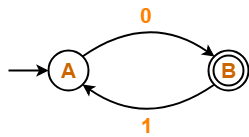## Solution-

### Step-01:

• Initial state A has an incoming edge.
• So, we create a new initial state qi.

The resulting DFA is-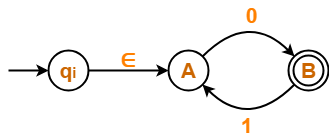### Step-02:

• Final state B has an outgoing edge.
• So, we create a new final state qf.

The resulting DFA is-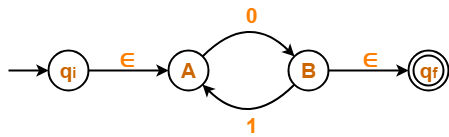### Step-03:

Now, we start eliminating the intermediate states.

First, let us eliminate state A.

• There is a path going from state qi to state B via state A.
• So, after eliminating state A, we put a direct path from state qi to state B having cost ∈.0 = 0
• There is a loop on state B using state A.
• So, after eliminating state A, we put a direct loop on state B having cost 1.0 = 10.

Eliminating state A, we get-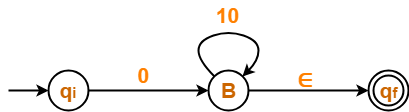### Step-04:

Now, let us eliminate state B.

• There is a path going from state qi to state qf via state B.
• So, after eliminating state B, we put a direct path from state qi to state qf having cost 0.(10)*.∈ = 0(10)*

Eliminating state B, we get-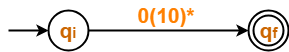From here,

 Regular Expression = 0(10)*

### NOTE-

In the above question,

• If we first eliminate state B and then state A, then regular expression would be = (01)*0.
• This is also the same and correct.

## Problem-02:

Find regular expression for the following DFA-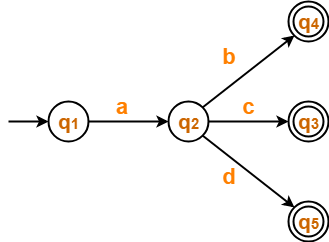## Solution-

### Step-01:

• There exist multiple final states.
• So, we convert them into a single final state.

The resulting DFA is-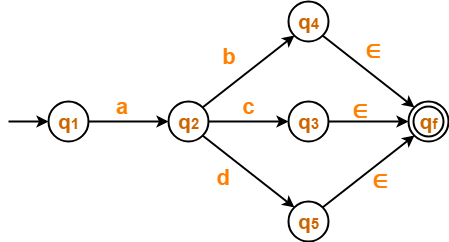### Step-02:

Now, we start eliminating the intermediate states.

First, let us eliminate state q4.

• There is a path going from state q2 to state qf via state q4.
• So, after eliminating state q4 , we put a direct path from state q2 to state qf having cost b.∈ = b.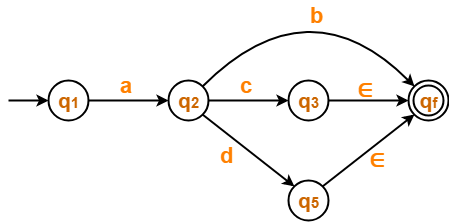### Step-03:

Now, let us eliminate state q3.

• There is a path going from state q2 to state qf via state q3.
• So, after eliminating state q3 , we put a direct path from state q2 to state qf having cost c.∈ = c.### Step-04:

Now, let us eliminate state q5.

• There is a path going from state q2 to state qf via state q5.
• So, after eliminating state q5 , we put a direct path from state q2 to state qf having cost d.∈ = d.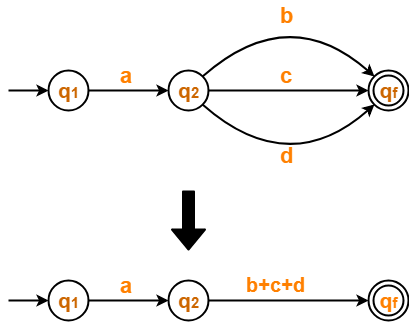### Step-05:

Now, let us eliminate state q2.

• There is a path going from state q1 to state qf via state q2.
• So, after eliminating state q2 , we put a direct path from state q1 to state qf having cost a.(b+c+d).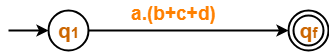From here,

 Regular Expression = a(b+c+d)

## Problem-03:

Find regular expression for the following DFA-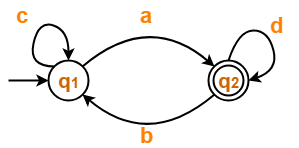## Solution-

### Step-01:

• Initial state q1 has an incoming edge.
• So, we create a new initial state qi.

The resulting DFA is-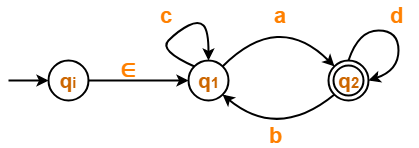### Step-02:

• Final state q2 has an outgoing edge.
• So, we create a new final state qf.

The resulting DFA is-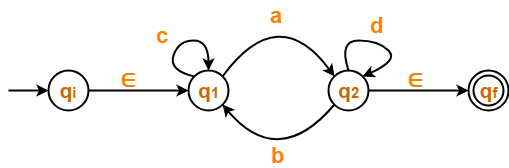### Step-03:

Now, we start eliminating the intermediate states.

First, let us eliminate state q1.

• There is a path going from state qi to state q2 via state q1 .
• So, after eliminating state q1, we put a direct path from state qi to state q2 having cost ∈.c*.a = c*a
• There is a loop on state q2 using state q1 .
• So, after eliminating state q1 , we put a direct loop on state q2 having cost b.c*.a = bc*a

Eliminating state q1, we get-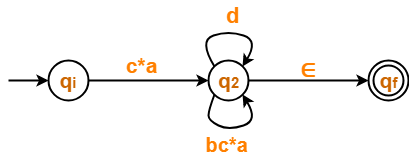### Step-04:

Now, let us eliminate state q2.

• There is a path going from state qi to state qf via state q2 .
• So, after eliminating state q2, we put a direct path from state qi to state qf having cost c*a(d+bc*a)*∈ = c*a(d+bc*a)*

Eliminating state q2, we get-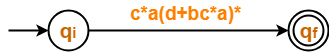From here,

 Regular Expression = c*a(d+bc*a)*

## Problem-04:

Find regular expression for the following DFA-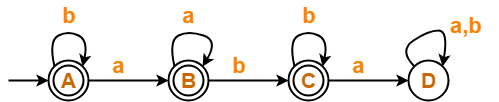## Solution-

### Step-01:

• State D is a dead state as it does not reach to any final state.
• So, we eliminate state D and its associated edges.

The resulting DFA is-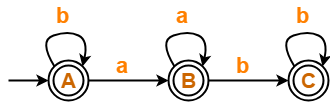### Step-02:

• Initial state A has an incoming edge (self loop).
• So, we create a new initial state qi.

The resulting DFA is-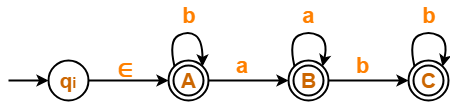### Step-03:

• There exist multiple final states.
• So, we convert them into a single final state.

The resulting DFA is-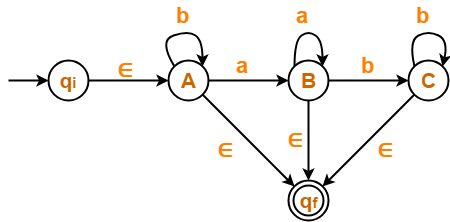### Step-04:

Now, we start eliminating the intermediate states.

First, let us eliminate state C.

• There is a path going from state B to state qf via state C.
• So, after eliminating state C, we put a direct path from state B to state qf having cost b.b*.∈ = bb*

Eliminating state C, we get-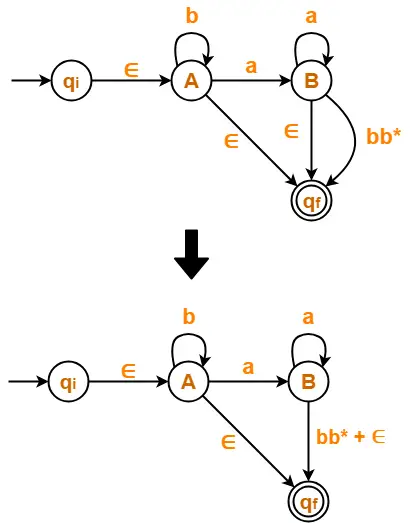### Step-05:

Now, let us eliminate state B.

• There is a path going from state A to state qf via state B.
• So, after eliminating state B, we put a direct path from state A to state qf having cost a.a*.(bb*+∈) = aa*(bb*+∈)

Eliminating state B, we get-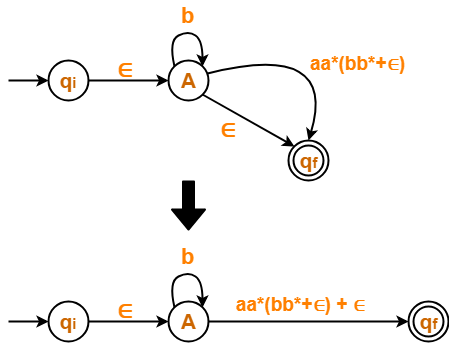### Step-06:

Now, let us eliminate state A.

• There is a path going from state qi to state qf via state A.
• So, after eliminating state A, we put a direct path from state qi to state qf having cost ∈.b*.(aa*(bb*+∈)+∈) = b*(aa*(bb*+∈)+∈)

Eliminating state A, we get-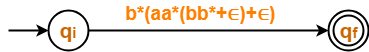From here,

 Regular Expression = b*(aa*(bb*+∈)+∈)

We know, bb* + ∈ = b*

So, we can also write-

 Regular Expression = b*(aa*b*+∈)

## Problem-05:

Find regular expression for the following DFA-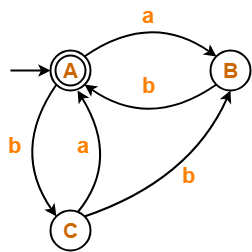## Solution-

### Step-01:

• Since initial state A has an incoming edge, so we create a new initial state qi.
• Since final state A has an outgoing edge, so we create a new final state qf.

The resulting DFA is-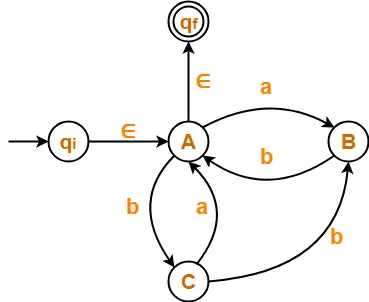### Step-02:

Now, we start eliminating the intermediate states.

First, let us eliminate state B.

• There is a path going from state C to state A via state B.
• So, after eliminating state B, we put a direct path from state C to state A having cost b.b = bb.
• There is a loop on state A using state B.
• So, after eliminating state B, we put a direct loop on state A having cost a.b = ab.

Eliminating state B, we get-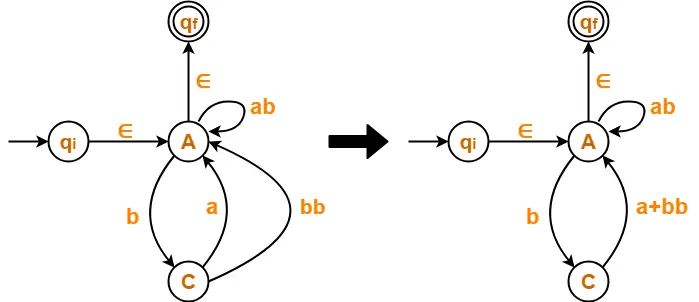### Step-03:

Now, let us eliminate state C.

• There is a loop on state A using state C.
• So, after eliminating state C, we put a direct loop on state A having cost b.(a+bb) = b(a+bb)

Eliminating state C, we get-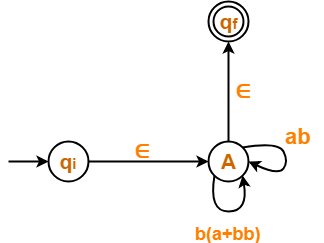### Step-04:

Now, let us eliminate state A.

• There is a path going from state qi to state qf via state A.
• So, after eliminating state A, we put a direct path from state qi to state qf having cost ∈.(ab + b(a+bb))*∈ = (ab + b(a+bb))*

Eliminating state A, we get-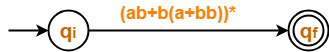From here,

 Regular Expression = (ab + b(a+bb))*

## Problem-06:

Find regular expression for the following DFA-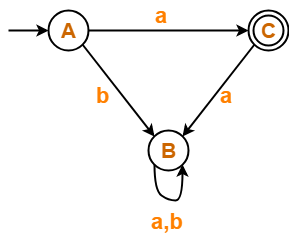## Solution-

• State B is a dead state as it does not reach to the final state.
• So, we eliminate state B and its associated edges.

The resulting DFA is-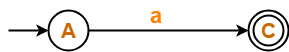From here,

 Regular Expression = a

## Problem-07:

Find regular expression for the following DFA-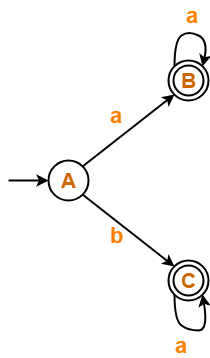## Solution-

### Step-01:

• There exist multiple final states.
• So, we create a new single final state.

The resulting DFA is-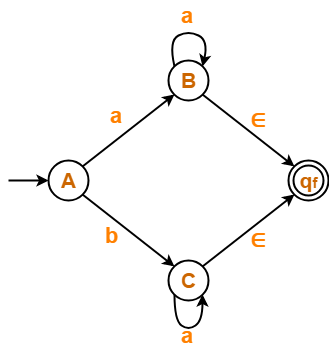### Step-02:

Now, we start eliminating the intermediate states.

First, let us eliminate state B.

• There is a path going from state A to state qf via state B.
• So, after eliminating state B, we put a direct path from state A to state qf having cost a.a*.∈ = aa*.

Eliminating state B, we get-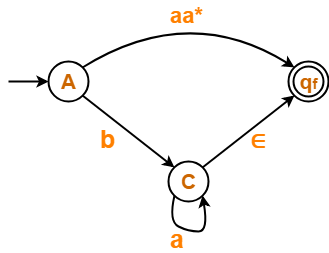### Step-03:

Now, let us eliminate state C.

• There is a path going from state A to state qf via state C.
• So, after eliminating state C, we put a direct path from state A to state qf having cost b.a*.∈ = ba*.

Eliminating state C, we get-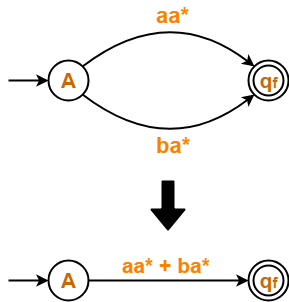From here,

 Regular Expression = aa* + ba*

To gain better understanding about Converting DFA to Regular Expression,

Watch this Video Lecture

Next Article- Arden’s Theorem

Get more notes and other study material of Theory of Automata and Computation.

Watch video lectures by visiting our YouTube channel LearnVidFun.

SummaryArticle Name
DFA to Regular Expression | Examples
Description
DFA to Regular Expression- The methods to convert DFA to regular expression are- Arden's Method and State Elimination Method. Convert DFA to a Regular Expression Using State Elimination Method. DFA to Regular Expression Conversion Exercises.
Author
Publisher Name
Gate Vidyalay
Publisher Logo